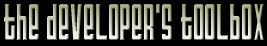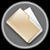This section of the archives stores flipcode's complete Developer Toolbox collection, featuring a variety of mini-articles and source code contributions from our readers.Fast log() Function   Submitted byHere is a code snippet to replace the slow log() function... It just performs an approximation, but the maximum error is below 0.007. Speed gain is about x5, and probably could be increased by tweaking the assembly code.

The function is based on floating point coding. It's easy to get floor (log2(N)) by isolating exponent part. We can refine the approximation by using the mantissa. This function returns log2(N) :

 ```inline float fast_log2 (float val) { int * const exp_ptr = reinterpret_cast

The line (1) computes 1+log2(m), m ranging from 1 to 2. The proposed formula is a 3rd degree polynomial keeping first derivate continuity. Higher degree could be used for more accuracy. For faster results, one can remove this line, if accuracy is not the matter (it gives some linear interpolation between powers of 2).

Now we got log2(N), we have to multiply it by ln(2) to get the natural log :

 ```inline float fast_log (const float &val) { return (fast_log2 (val) * 0.69314718f); } ```

-- Laurent

The zip file viewer built into the Developer Toolbox made use of the zlib library, as well as the zlibdll source additions.